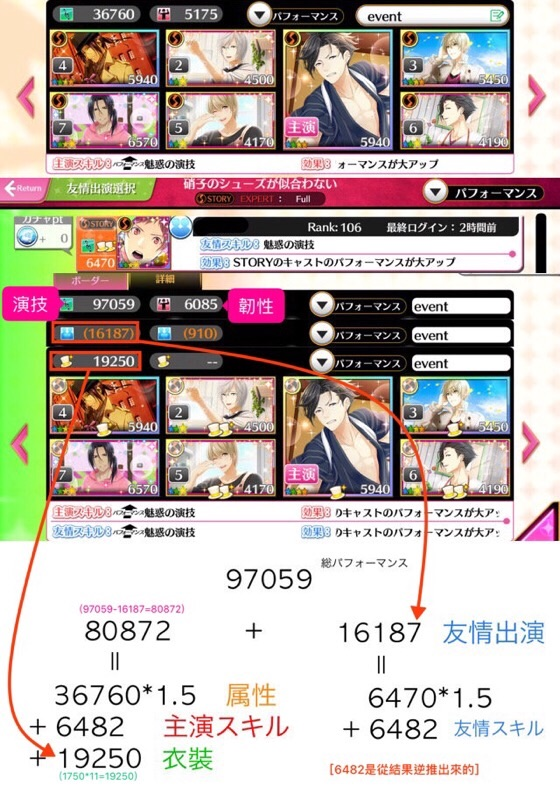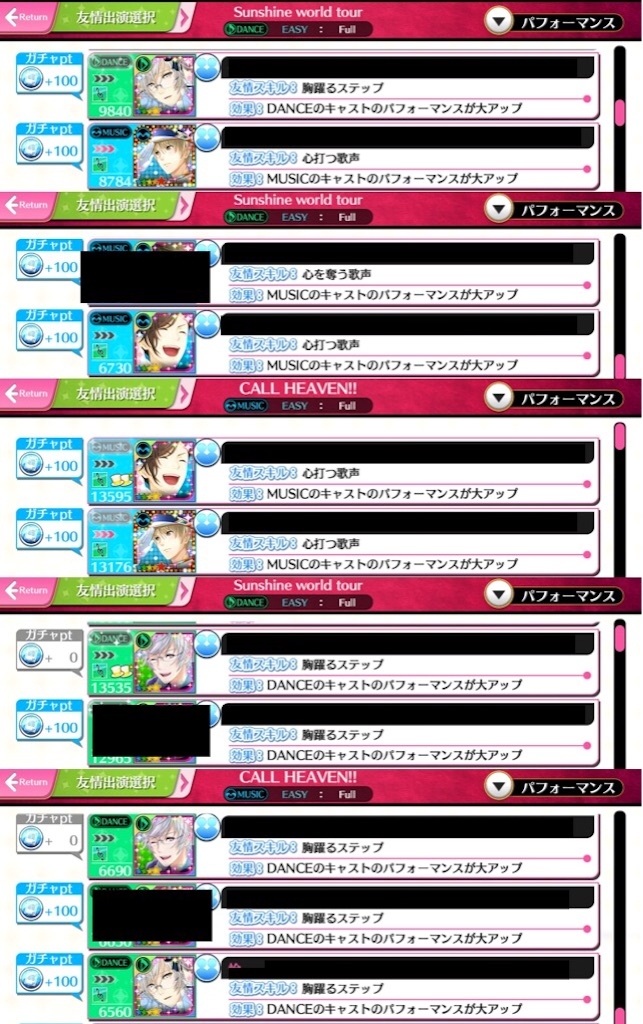# 夢色キャスト 隊伍、分數、活動點數

（2018/3/13 更新）

## 隊伍數值• 加成後的總數值
• （有括號）朋友的卡+客演加成
• （帽子）衣裝加成

（朋友的主演在分數條裡沒有顯示顏色，直接加在友情出演裡）

• 大UP×0.149 (15%)
• 中UP×0.119 (12%)

（小數點捨去的樣子？）## 分數

• 綠色：跟黃色一樣
• 藍色：基本分×1.2×2 （長按有前後）
• 紅色：基本分×1.1×2 （同時按有兩顆）

（很久以前算的，不一定準確只是給個概念）

• Combo

Combo數愈大，加成愈大。詳細加成其實我沒有算清楚（喂）

• 卡片技能

（Tension Bonus記得使用！）

• 親密度

## 活動pt

（原文張貼於 FB社團 夢色卡司中文版交流區）

• 普通 18
• 困難 33
• 專家 50

→ 非活動曲打C分就是拿基本pt

※ 以下計算過程的小數點後都直接進位

• S Rank (×1.3) ⋯ 計算 = 基本pt ×1.3 = A ⋯ 後面數字 = (A-基本)
• S Rank突破 (× ) ⋯ 計算 = 基本pt ×突破= B ⋯ 後面數字 = B
• 樂曲 (×1.5) ⋯ 計算 = (A+B)×1.5 = C ⋯ 後面數字 = (C-A-B)
• 名次 (× ) ⋯ 計算 = (A+B)×名次 = C ⋯ 後面數字 = (C-A-B)
• 演員 (× ) ⋯ 計算 = C×演員 = D ⋯ 後面數字 = D
• 最終獲得pt = C + D

• 活動形式不同，基本pt也會不同

→ 中括號裡是 [活動曲 無特效] 的pt

※ 圖片原張貼於 FB社團 夢色卡司中文版交流區

（只是要說明那些數字不是隨便亂寫的！）

• ① 分數的「S Rank突破」
• ② 活動特效

§